• 一维数组不知道他的分布情况下。对数的频率分布直方图尽可能拟合。 数组我们用matlab自带的函数来生成。频数统计区间默认划 首先生成一个服从（0，0.5^2）的高斯分布随机产生10000个数 x=normrnd(0,1,1,10000);%产生...
本文解决的用matlab实现数组的概率分布函数拟合。
一维数组不知道他的分布情况下。对数的频率分布直方图尽可能拟合。
数组我们用matlab自带的函数来生成。频数统计区间默认划
首先生成一个服从（0，0.5^2）的高斯分布随机产生10000个数
x=normrnd(0,1,1,10000);%产生一个[10000*1]的矩阵按照高斯（0，1^2）分布
plot(x,'*')%R = normrnd(Mu, Sigma, m, n)产生服从N（Mu，Sigma^2）分布的m行n列的随机数组R

数组x为一个【10000*1】一维向量。下图将这10000个点画出。下一步利用频数分布直方图，画出频率直方图。尽可能地将区间划小可以表示成统计形式而不是类似条形图。这里d是统计的区间

x=normrnd(0,1,1,10000);%产生一个[100*1]的矩阵按照高斯（0，0.5^2）分布
%% 做出概率分布图
d=100;%划分100分布区间用于统计频数小于原始数据就行。
b=min(x):(max(x)-min(x))/(d-1):max(x);
pn= histc(x,b);%频率分布区间直方图，x为输入数组，d为统计区间的个数
p=pn./((max(x)-min(x))/(d-1)*length(x));%频数/（组距*总数）=频率

plot(b,pn)
plot(b,p)上图是频数图。下图是频率图；对坐标的处理是影响结果的关键**这里有个问题，就是频率=频数/组距/总数。组距是人为设定的所以概率必然与真实的概率有一些差别。我们只能在这里期望这是选择了一个合适的统计频数的区间了！！！**所以这里只能期望是大数据量的拟合数组了。
之后使用多项式拟合，多项式的系数可以修改。生成w就是系数矩阵。
保存w，使用y=polyval(w,x).就是y关于x的拟合的概率密度函数。

W=polyfit(b,p,10);%多项式拟合的项数为10+1(bias)次，这里可以调整项数
xi=min(x):(max(x)-min(x))/(d-1):max(x);
yi=polyval(W,xi);
figure
plot(xi,yi,b,p,'r*');
figure
plot(b,p,'r*');
figure
plot(xi,yi)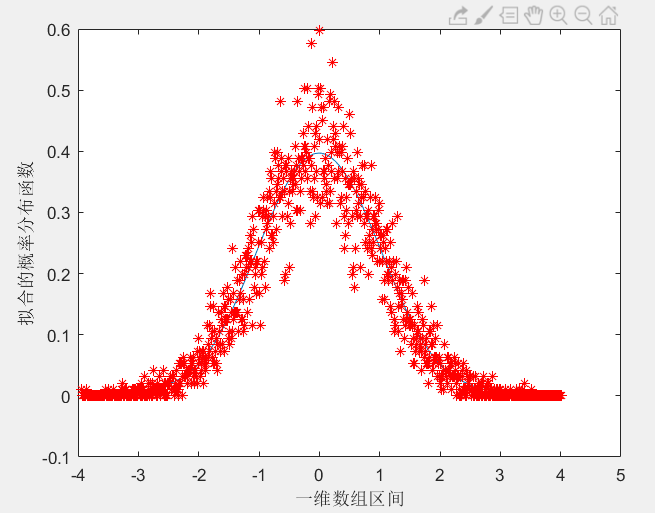最后我求解一下x=0时候的概率值。
y=polyval(W,0)我试着带入高斯单变量分布的数学公式。正太分布的数学公式：
N~（0，1）x=0;u=0;o=1;pi=3.14;
得到f(0)=0.399.
验证后：这条拟合概率分布函数近似实际还是可以使用的。
整体函数如下：
clear
%% 创建数据
x=normrnd(0,1,1,10000);%产生一个[10000*1]的矩阵按照正太（0，1）分布
plot(x,'*')

%% 画出数据集的频率分布图
d=100;%划分100分布区间用于统计频数小于原始数据就行。
X=min(x):(max(x)-min(x))/(d-1):max(x);%X划分区间的数组
pn= histc(x,X);%频率分布区间直方图，x为输入数组，d为统计区间的个数
P=pn./((max(x)-min(x))/(d-1)*length(x));%频数/（组距*总数）=频率
plot(X,P)

%% 拟合多项式函数
W=polyfit(X,P,10);%多项式拟合的项数为10+1(bias)次，这里可以调整项数
xi=min(x):(max(x)-min(x))/(d-1):max(x);
yi=polyval(W,xi);
figure
plot(xi,yi,X,P,'r*');
xlabel('一维数组区间')
ylabel('拟合的概率分布函数')

%% 函数验证
P_nihe=polyval(W,0)
P_shiji=1/sqrt(6.282)

如果有谁能够解决直方图划分区间，也就是自动划分合适区间大小（或者是自动计算出直方图统计分成几段）而不是（matlab中默认的10个区间）。统计频数问题的请留言。


展开全文• 高斯分布概率密度函数数据集实战优化坐标轴与图像优化图像再次优化 概率密度函数 大家肯定都有听说过正态分布，其实正态分布只是概率密度分布的种，正态分布的概率密度函数均值为μ ，标准差σ是高斯函数的...
二维高斯分布概率密度函数数据集实战优化坐标轴与图像优化图像再次优化
概率密度函数
大家肯定都有听说过正态分布，其实正态分布只是概率密度分布的一种，正态分布的概率密度函数均值为μ ，标准差σ是高斯函数的一个实例：
$f(x ; \mu, \sigma)=\frac{1}{\sigma \sqrt{2 \pi}} \exp \left(-\frac{(x-\mu)^{2}}{2 \sigma^{2}}\right)$
在一维上只有x一个变量，μ 均值，σ标准差。
正态分布具有两个参数μ和σ的连续型随机变量的分布，第一
参数μ是服从正态分布的随机变量的均值，第二个参数σ^2是此随机变量的方差，所以正态分布记作N（μ,σ2）。
实际工作中，正态曲线下横轴上一定区间的面积反映该区间的例数占总例数的百分比，或变量值落在该区间的概率。
因此一维的概率密度分布即正态分布，很好的表示数据在哪个区间集中，使我们对整体数据有一个大概的把握。
本文的重点在于二维概率密度函数：
$f(x, y)=\left(2 \pi \sigma_{1} \sigma_{2} \sqrt{1-\rho^{2}}\right)^{-1} \exp \left[-\frac{1}{2\left(1-\rho^{2}\right)}\left(\frac{\left(x-\mu_{1}\right)^{2}}{\sigma_{1}^{2}}-\frac{2 \rho\left(x-\mu_{1}\right)\left(y-\mu_{2}\right)}{\sigma_{1} \sigma_{2}}+\frac{\left(y-\mu_{2}\right)^{2}}{\sigma_{2}^{2}}\right)\right]$
因为生活中的很多数据都是高维度的，从简单的二维说起。二维上的数据生活中有很多：身高和体重，血压和血脂等等。如果能够像一维正态分布那样做出图像来看，就十分直观，而本文就是介绍如何作二维概率密度函数的图像。
数据集
首先贴上数据集：
链接：https://pan.baidu.com/s/1RJCwi4-8_hByY6-rCepJgQ
提取码：88ew
数据是截至4.25日的重点国家新冠肺炎感染人数，有中国、美国、法国、意大利等。
本文采取的是中国和意大利进行对比分析。
import numpy as np
import matplotlib.pyplot as plt
import math
import mpl_toolkits.mplot3d
import math
import pandas as pd

x = data.iloc[:,1]
y = data.iloc[:,7]
x = x.values
y = y.values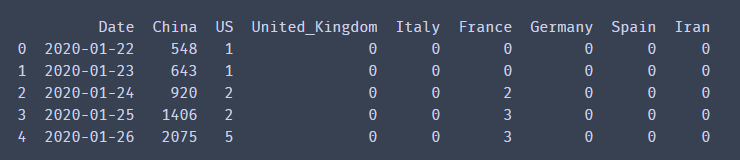实战
首先根据公式我们先把2个维度的均值和方差分别计算出来，以及公式中需要的相关系数。

u1 = x.mean()
u2 = y.mean()
o1 = x.std()
o2 = y.std()
from scipy.stats import pearsonr
p = pearsonr(x, y)
print(u1, u2, o1, o2, p)

# 输出:(r, p)
# r:相关系数[-1，1]之间
# p:相关系数显著性

相关系数也就是皮尔逊系数，把2个维度数据给入后，会输出相关系数和相关系数显著性。
相关系数取值范围是(-1，1)，越接近1则说明越相关。不过我们也不能说中国感染人数和西班牙感染人数相关，这里更确切地解释应该是感染人数的趋势比较。
X, Y = np.meshgrid(x, y)
z = (1/(2*math.pi*o1*o2*pow(1-pow(p,2),0.5)))*np.exp(-1/(2*(1-p*p))*(((X-u1)*(X-u1))/(o1*o1)-2*p*(X-u1)*(Y-u2)/(o1*o2)+(Y-u2)*(Y-u2)/(o2*o2)))

这里X,Y是对原始数据进行网格化，其实就相当于最后成果图的横纵坐标，只是转换一下得以输入作图。
z就是上文的二维密度函数用python来表达了。比较麻烦，注意里面有上面算出的2个维度的均值，方差和皮尔逊系数。
plt.rcParams['font.sans-serif'] = ['KaiTi']  # 用来正常显示中文字符
plt.rcParams['axes.unicode_minus'] = False
plt.figure(figsize=(10,10), dpi=300)
ax = plt.subplot(111, projection='3d')
ax.plot_surface(X, Y, z,
cmap='rainbow', alpha=0.9)
ax.set_xlabel('中国感染人数')
ax.set_ylabel('西班牙感染人数')
ax.set_zlabel('频率')
ax.set_title("二维高斯分布")

这就是很基础的一些画图设置了，相似的就不再赘述，重点 谈谈plot_surface。
plot_surface中的X，Y，z其实上文以及解释过了，就是相应的坐标和函数，那么cmap是什么呢，camp是颜色盘，值定位rainbow就是彩虹色，从下图就可以看出，数据越集中的地方，颜色就越深。这里还有一个颜色盘是coolwarm，不过个人感觉没rainbow好看，不妨小伙伴们试一试。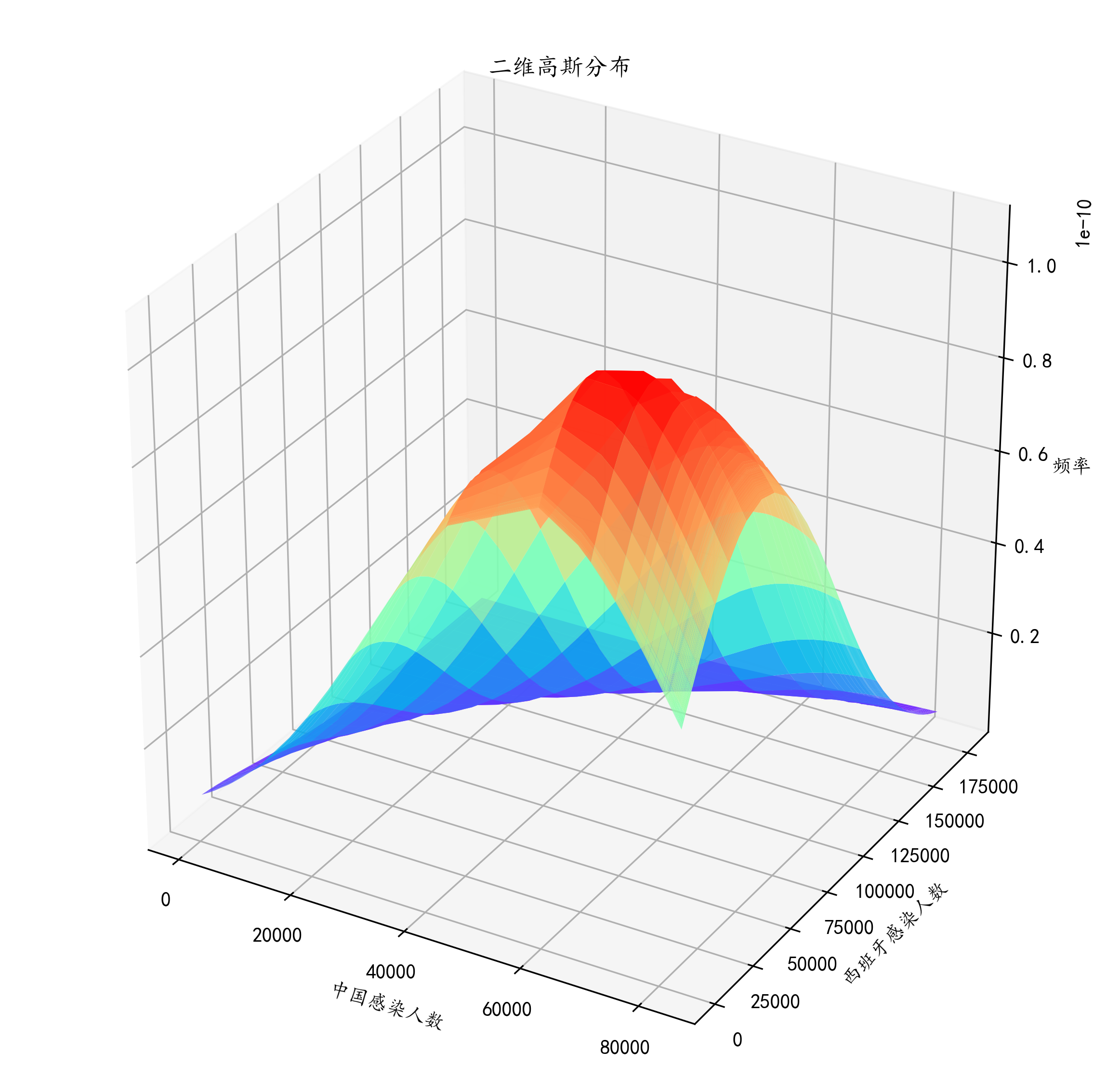到此我们就大概的画出了中国感染人数和西班牙感染人数在4.25之前的密度函数。
这个图我们看出，中国感染人数大概在4-5万就开始达到高峰，之后开始下降，而西班牙到了12万左右才开始下降。整个国家感染人数的增幅一目了然，对于整体数据的把握也有较好的认知。但这样似乎不太好看，而且到底高峰是不是在我说的那个数值呢，根据肉眼都不好判断。所以我们接下来进行优化。
优化
坐标轴与图像优化
plt.rcParams['font.sans-serif'] = ['KaiTi']  # 用来正常显示中文字符
plt.rcParams['axes.unicode_minus'] = False
plt.figure(figsize=(10,10), dpi=300)
ax = plt.subplot(111, projection='3d')
ax.plot_surface(X, Y, z, rstride=1, cstride=1,
cmap='rainbow', alpha=0.9)
ax.set_xlabel('中国感染人数', fontsize=15)
ax.set_ylabel('西班牙感染人数', fontsize=15)
ax.set_zlabel('频率', fontsize=15)
ax.set_title("二维高斯分布", fontsize=25, y=1.02)

ax.set_xticks(np.arange(0,100000,20000))
ax.set_yticks(np.arange(0,200000,40000))

可能一眼还没看出来。我来讲解一下。博主在plot_surface里面加了 rstride=1, cstride=1,这两个参数有什么作用？相当于步长。这么理解吧，这个颜色实际上是由无数个点组成的，但是实际上就像房子顶上的瓦片一样，如果瓦片比较大，那么房顶面积一定，瓦片就用的少，就像上图一样显得一块一块的，非常大，不过不平滑。而下图呢，加入 rstride=1, cstride=1就相当于定制了瓦片长宽，瓦片比较小那么看起来就舒服，颜色过渡得比上面那个自然多。
把标题和坐标轴都修改一下，title的x，y参数是调位置的，如何使用的话小伙伴们多试几个值就明白了。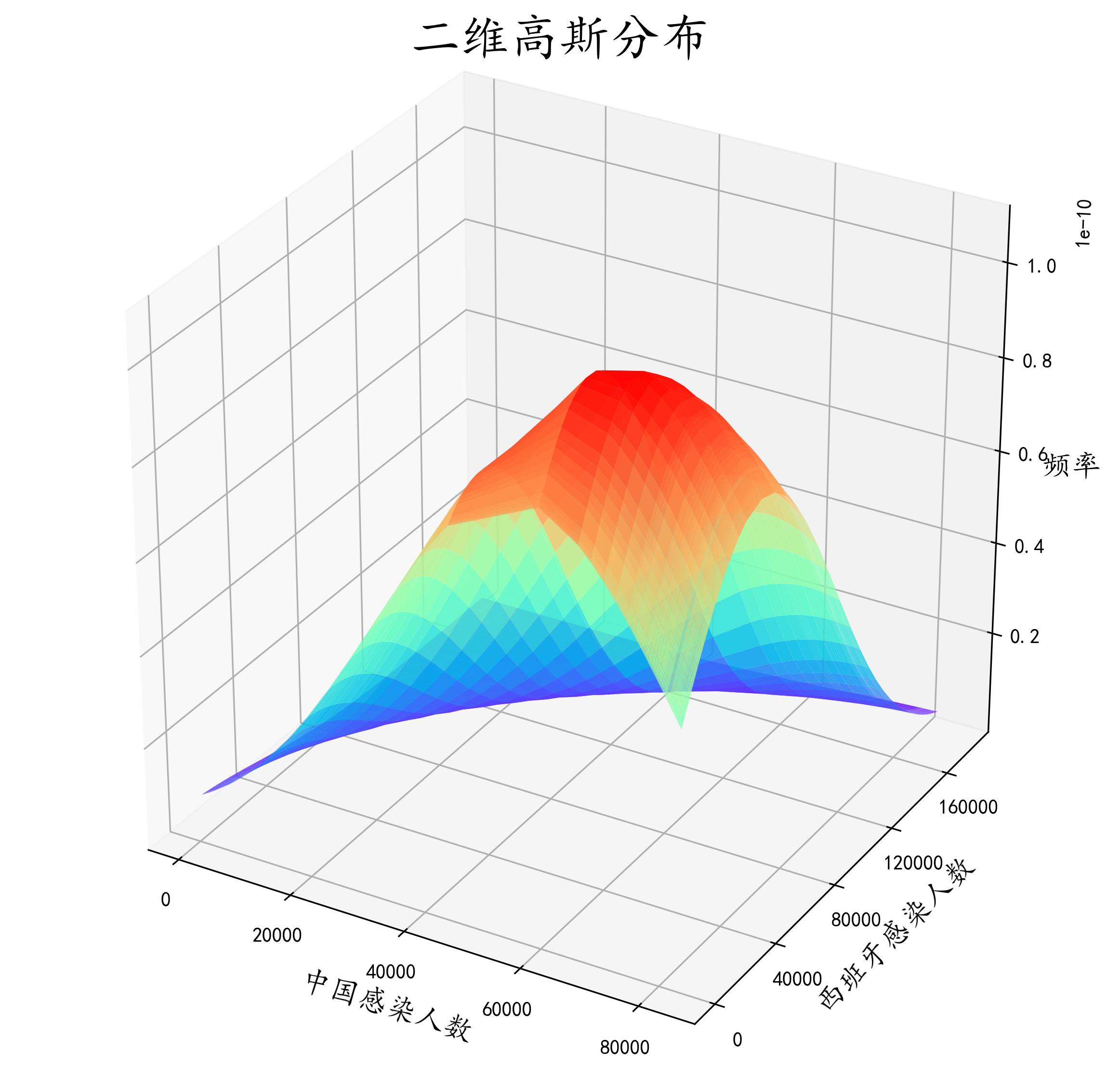图像再次优化
plt.rcParams['font.sans-serif'] = ['KaiTi']  # 用来正常显示中文字符
plt.rcParams['axes.unicode_minus'] = False
plt.figure(figsize=(10,10), dpi=300)
ax = plt.subplot(111, projection='3d')
ax.plot_surface(X, Y, z, rstride=1, cstride=1,
cmap='rainbow', alpha=0.9)
ax.set_xlabel('中国感染人数', fontsize=15)
ax.set_ylabel('西班牙感染人数', fontsize=15)
ax.set_zlabel('频率', fontsize=15)
ax.set_title("二维高斯分布", fontsize=25, y=1.02)

ax.set_xticks(np.arange(0,100000,20000))
ax.set_yticks(np.arange(0,200000,40000))
ax.contour(X, Y, z, 15, zdir = 'z', offset = 0, cmap = plt.get_cmap('rainbow'))
ax.w_xaxis.set_pane_color((135/255, 206/255, 250/255, 0.3))
ax.w_yaxis.set_pane_color((135/255, 206/255, 250/255, 0.3))
ax.w_zaxis.set_pane_color((135/255, 206/255, 250/255, 0.3))


作图一方面为了好看，一方面是对数据整体把握更加直观，这里加了ax.w_xaxis.set_pane_color这个方法是对x平面进行上色，个人感觉更好看吧。里面的参数是rgba。
细心的小伙伴已经发现了，这个图比上面的多了好多等高线。这些等高线是这个密度函数在xoy平面的投影，能够更直观的看出到底数据的高峰是在哪。我们直观看出，中国感染人数到达高峰是在6w人左右，而西班牙也是在6w人左右，这和我们前面目测估计的有一点误差。所以ax.contour这个方法将密度函数投影到平面来，更细致的观察数据的分布。其中ax.contour中
15代表是有多少条等高线，zdir=z表示投影到z=？这个平面，而？的数值就是由offset表示，这里显然投影到z=0平面，camp也是和上文意思差不多是颜色盘，彩虹色的。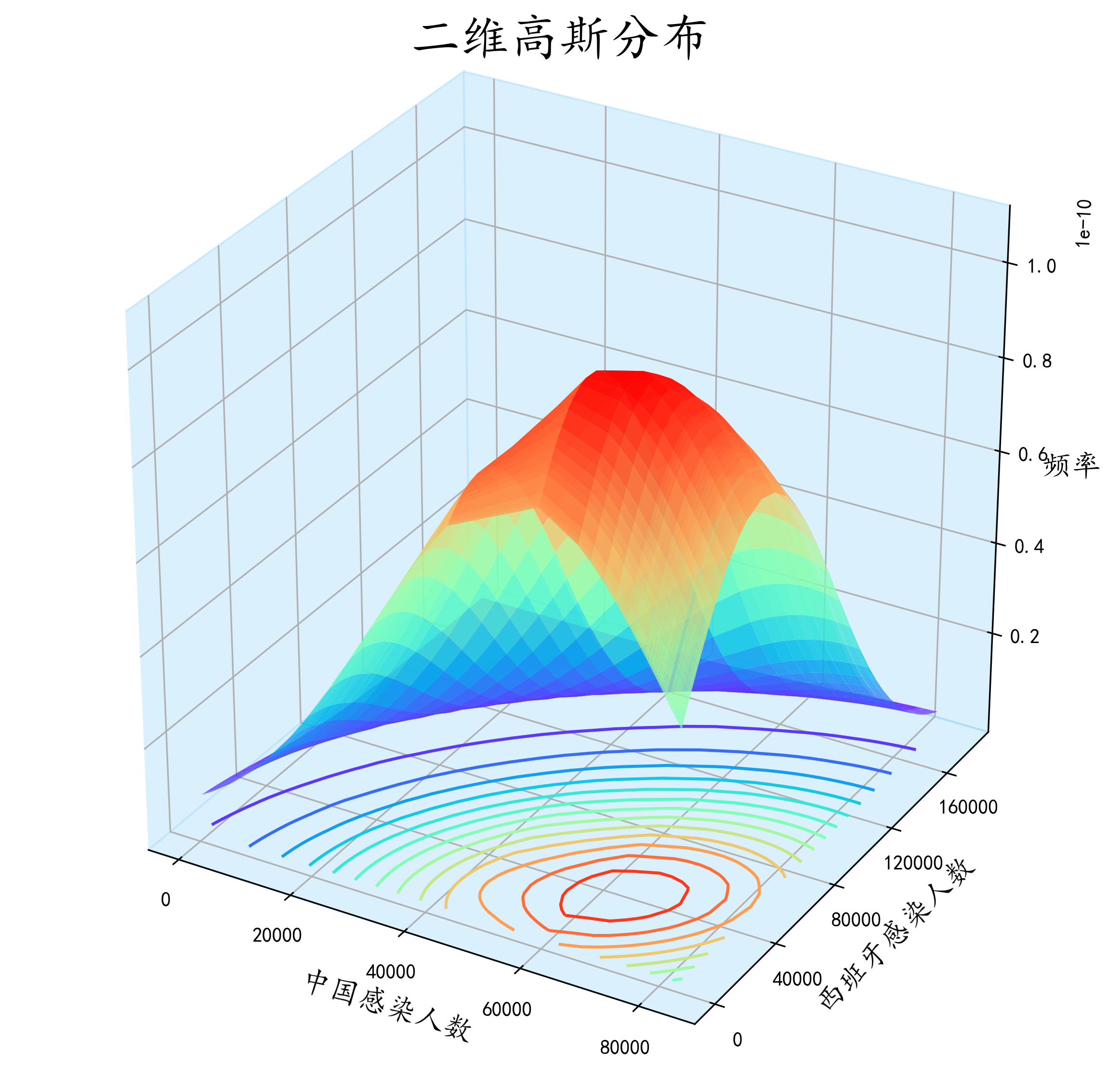效果还是很直观的。


展开全文数据分析 python 机器学习
• 之前的博客中已经提到，贝叶斯决策的基础是概率密度函数的估计，即根据一定的训练样本来估计统计决策中用到的先验概率P(wi)P(w_i)P(wi​)和类条件概率密度p(x∣wi)p(x|w_i)p(x∣wi​)。 概率密度函数的估计分为参数...
之前的博客中已经提到，贝叶斯决策的基础是概率密度函数的估计，即根据一定的训练样本来估计统计决策中用到的先验概率$P(w_i)$和类条件概率密度$p(x|w_i)$。
概率密度函数的估计分为参数估计和非参数估计。
极大似然估计
极大似然估计属于一种典型的参数估计法。
在最大似然估计(maximum likelihood estimation)中，我们做以下基本假设：

待估计参数$\theta$是确定但未知的量；
每类中的样本满足独立同分布条件；
类条件概率密度$p(x|w_i)$具有某种确定的函数形式，只是其中的参数未知；
不同类别的参数是独立的。

设样本集包含$N$个样本，即$S=\{x_1,x_2,...,x_N\}$，由于样本是独立地从$p(x|\theta)$中抽取的，所以在概率密度为$p(x|\theta)$时获得样本集$S$的概率即出现$S$中的各个样本的联合概率是：
$l(\theta)=p(S|\theta)=p(x_1,x_2,...,x_N|\theta)=\prod_{i=1}^np(x_i|\theta)$
这个概率反映了在概率密度函数的参数是$\theta$时，得到这组样本的概率。上述公式称作参数$\theta$相对于样本集$S$的似然函数(lielihood function)。
对于未知参数$\theta$，我们从一次抽样中得到了$N$个样本，求解所抽取的这组样本来自哪个密度函数（$\theta$取什么值）的可能性最大的过程就是最大似然估计。为了方便分析，还可以定义对数似然函数：
$H(\theta)=\ln l(\theta)=\ln \prod_{i=1}^np(x_i|\theta)=\sum_{i=1}^n\ln p(x_i|\theta)$
很显然，使对数似然函数最大的$\theta$值也使似然函数最大。
最大似然估计的求解
似然函数的未知量只有$\theta$，在该函数满足连续、可微的条件下，如果$\theta$是一维变量，其最大似然函数估计量就是如下微分方程的解：$\frac{dl(\theta)}{d\theta}=0$。当$\theta$是多维变量时，求解过程需要对$\theta$的每一维分别求偏导，来对似然函数求梯度并令其等于零。在某些情况下，似然函数可能有多个极值，因此可能有多个解，其中使似然函数最大的那个解才是最大似然估计量。
正态分布下的最大似然估计，有两个参数（均值$\mu和方差\sigma^2$）为未知，分布对两个未知参数求偏导，可以解得：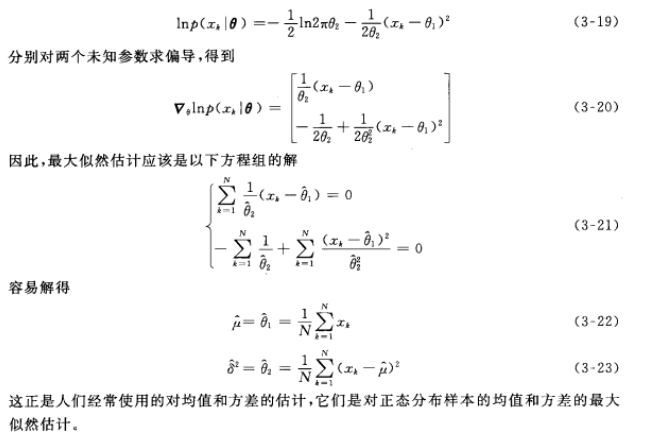其实就是各类训练样本的均值和方差。
贝叶斯估计
贝叶斯估计是另一类主要的参数估计方法，其结果在很多情况下与最大似然法相同或几乎相同。与极大似然估计相比，贝叶斯估计的优势不但在于使用样本中提供的信息进行估计，而且能够很好地把参数估计的先验知识融合进来，并且能够根据数据量大小和先验知识的确定程度来调和两部分信息的相对贡献，这在很多实际问题中是非常有用的。
最大似然估计是把待估计的参数当做未知但固定的量，要做的是根据观察数据估计这个量的取值；而贝叶斯估计则把待估计的参数本身也看作是随机变量，要做的是根据观测数据对参数的分布进行估计，除了观测数据外，还可以考虑参数的先验分布。
注：如无特殊说明，以上大部分内容为摘选自张学工所著《模式识别》。


展开全文极大似然估计
• 概率密度函数是概率论中的核心概念之，用于描述连续型随机变量所服从的概率分布。 从随机事件说起 回忆我们在学习概率论时的经历，随机事件是第个核心的概念，它定义为可能发生也可能不发生的事件，因此是否发生...
1. 概率函数
概率函数，就是用函数的形式来表达概率。
$p_i=P(X=a_i)(i=1,2,3,4,5,6)$
在这个函数里，自变量（X）是随机变量的取值，因变量（$p_i$）是取值的概率。这就叫啥，这叫用数学语言来表示自然现象！它就代表了每个取值的概率，所以顺理成章的它就叫做了X的概率函数。
从公式上来看，概率函数一次只能表示一个取值的概率。比如P（X=1）=1/6,这代表用概率函数的形式来表示，当随机变量取值为1的概率为1/6，一次只能代表一个随机变量的取值。
2. 概率分布
概率分布，就是概率的分布，这个概率分布还是讲概率的。我认为在理解这个概念时，关键不在于“概率”两个字，而在于“分布”这两个字。为了理解“分布”这个词，我们来看一张图。在很多教材中，这样的列表都被叫做离散型随机变量的“概率分布”。其实严格来说，它应该叫“离散型随机变量的值分布和值的概率分布列表”，这个名字虽然比“概率分布”长了点，但是对于我们这些笨学生来说，肯定好理解了很多。因为这个列表，上面是值，下面是这个取值相应取到的概率，而且这个列表把所有可能出现的情况全部都列出来了！
举个例子吧，一颗6面的骰子，有1，2，3，4，5，6这6个取值，每个取值取到的概率都为1/6。那么你说这个列表是不是这个骰子取值的”概率分布“？长得挺像的，上面是取值，下面是概率，这应该就是骰子取值的“概率分布”了吧！大错特错！少了一个最重要的条件！对于一颗骰子的取值来说，它列出的不是全部的取值，把6漏掉了!
这么一说你就应该明白概率分布是个什么鬼了吧。
3. 分布函数
说完概率分布，就该说说分布函数了。这个分布函数又是个简化版的东西！我真的很讨厌我们的教材中老是故弄玄虚，卖弄概念！你就老老实实的写成”概率分布函数“，让我们这些笨学生好理解一些不行吗？
看看下图中的分布律！这又是一个不统一叫法的丑恶典型！这里的分布律明明就是我们刚刚讲的“概率函数”，完全就是一个东西嘛！但是我知道很多教材就是叫分布律的。我们来看看图上的公式，其中的F(x)就代表概率分布函数啦。这个符号的右边是一个长的很像概率函数的公式，但是其中的等号变成了大于等于号的公式。你再往右看看，这是一个一个的概率函数的累加！发现概率分布函数的秘密了吗？它其实根本不是个新事物，它就是概率函数取值的累加结果！所以它又叫累积概率函数！其实，我觉得叫它累积概率函数还更好理解！！
概率函数和概率分布函数就像是一个硬币的两面，它们都只是描述概率的不同手段！
4. 概率密度函数
概率密度函数是概率论中的核心概念之一，用于描述连续型随机变量所服从的概率分布。
4.1 从随机事件说起
研究一个随机变量，不只是要看它能取哪些值，更重要的是它取各种值的概率如何！
回忆我们在学习概率论时的经历，随机事件是第一个核心的概念，它定义为可能发生也可能不发生的事件，因此是否发生具有随机性。例如，抛一枚硬币，可能正面朝上，也可能反面朝上，正面朝上或者反面朝上都是随机事件。掷骰子，1到6这6种点数都可能朝上，每种点数朝上，都是随机事件。4.2 整数集与实数集
高中时我们学过集合的概念，并且知道整数集是z，实数集是R。对于有限集，可以统计集合中元素的数量即集合的基数（cardinal number，也称为集合的势cardinality）。对于无限集，元素的个数显然是无穷大，但是，都是无穷大，能不能分个三六九等呢？
回忆微积分中的极限，对于下面的极限：虽然当x趋向于正无穷的时候，x和exp(x)都是无穷大，但它们是有级别的，在exp(x)面前，x是小巫见老巫。
同样的，对于整数集和实数集，也是有级别大小的。任意两个整数之间，如1与2之间，都密密麻麻的分布着无穷多个实数，而且，只要两个实数不相等，不管它们之间有多靠近，如0.0000001和0.0000002，在它们之间还有无穷多个实数。在数轴上，整数是离散的，而实数则是连续的，密密麻麻的布满整个数轴。因此，实数集的元素个数显然比整数要高一个级别。
4.3 随机变量
变量是我们再熟悉不过的概念，它是指一个变化的量，可以取各种不同的值。随机变量可以看做是关联了概率值的变量，即变量取每个值有一定的概率。例如，你买彩票，最后的中奖金额x就是一个随机变量，它的取值有3种情况，以0.9的概率中0元，0.09的概率中100元，0.01的概率中1000元。变量的取值来自一个集合，可以是有限集，也可以是无限集。对于无限集，可以是离散的，也可以是连续的，前者对应于整数集，后者对应于实数集。
4.3.1 离散型随机变量
随机变量是取值有多种可能并且取每个值都有一个概率的变量。它分为离散型和连续型两种，离散型随机变量的取值为有限个或者无限可列个（整数集是典型的无限可列），连续型随机变量的取值为无限不可列个（实数集是典型的无限不可列）。4.3.2 连续型随机变量
把分布表推广到无限情况，就可以得到连续型随机变量的概率密度函数。此时，随机变量取每个具体的值的概率为0，但在落在每一点处的概率是有相对大小的，描述这个概念的，就是概率密度函数。你可以把这个想象成一个实心物体，在每一点处质量为0，但是有密度，即有相对质量大小。在概率论和统计学中，拉普拉斯是一种连续概率分布。由于它可以看做是俩个不同位置的指数分布背靠背拼在一起，所以它也叫做双指数分布。如果随机变量的概率密度函数分布为：那么他就是拉普拉斯分布。u为位置参数，b>0是尺度参数。与正态分布相比，正态分布是用相对于u平均值的差的平方来表示，而拉普拉斯概率密度用相对于差的绝对值来表示。因此，拉普拉斯的尾部比正态分布更加平坦。概率密度函数用数学公式表示就是一个定积分的函数，定积分在数学中是用来求面积的，而在这里，你就把概率表示为面积即可！左边是F(x)连续型随机变量分布函数画出的图形，右边是f(x)连续型随机变量的概率密度函数画出的图像，它们之间的关系就是，概率密度函数是分布函数的导函数。
两张图一对比，你就会发现，如果用右图中的面积来表示概率，利用图形就能很清楚的看出，哪些取值的概率更大！这样看起来是不是特别直观，特别爽！！所以，我们在表示连续型随机变量的概率时，用f(x)概率密度函数来表示，是非常好的！
但是，可能读者会有这样的问题：
Q：概率密度函数在某一点的值有什么意义？
A：比较容易理解的意义，某点的 概率密度函数 即为 概率在该点的变化率(或导数)。很容易误以为 该点概率密度值 为 概率值.
比如: 距离(概率)和速度(概率密度)的关系.某一点的速度, 不能以为是某一点的距离，没意义,因为距离是从XX到XX的概念，所以, 概率也需要有个区间.
这个区间可以是x的邻域（可以无限趋近于0）。对x邻域内的f（x）进行积分，可以求得这个邻域的面积，就代表了这个邻域所代表这个事件发生的概率。
4.4 期望E(X)与方差Var(X)
随机变量(Random Variable)X是一个映射，把随机试验的结果与实数建立起了一一对应的关系。而期望与方差是随机变量的两个重要的数字特这。
期望(Expectation, or expected value)是度量一个随机变量取值的集中位置或平均水平的最基本的数字特征；
方差(Variance)是表示随机变量取值的分散性的一个数字特征。 方差越大，说明随机变量的取值分布越不均匀，变化性越强；方差越小，说明随机变量的取值越趋近于均值，即期望值。4.4.1 期望和方差的运算性质
4.4.1.1 期望运算性质4.4.1.2 方差的运算性质4.4.1.3 期望与方差的联系4.4.2 协方差4.4.2.1 协方差的运算性质4.4.3 相关系数
4.4.3.1 定义
相关系数通过方差和协方差定义。两个随机变量的相关系数被定义为：4.4.3.2 性质
1、有界性
相关系数的取值范围为-1到1，其可以看成是无量纲的协方差。
2、统计意义
值越接近1，说明两个变量正相关性（线性）越强，越接近-1，说明负相关性越强，当为0时表示两个变量没有相关性。5. 常见概率分布
5.1 均匀分布（Uniform Distribution）5.2 伯努利分布（Bernoulli Distribution）5.3 二项分布（Binomial Distribution）
二项分布(Binomial distribution)是n重伯努利试验成功次数的离散概率分布。从定义可以看出，伯努利分布是二项分布在n=1时的特例5.4 负二项分布（Negative Binomial Distribution）5.5 几何分布（Geometric Distribution）
假定我们有一系列伯努利试验，其中每一个的成功概率为$p$，失败概率为$q=1-p$。在获得一次成功前要进行多次试验？
注意，这里的随机变量的概率分布就是一种几何分布。具体如下：几何分布的概率分布图如下，见之会有更形象地认知。为什么单独把几何分布和二项分布单独列出，一方面其代表的概率试验的普适性，另一方面其期望和方差都是有特殊技巧。其实有意思的是，这里面的求解过程；但是本文不具体涉及了。因为像几何分布和二项分布这种可能要多写几章，当然是否连续写就不知道了。本着实用主义来。
一般简单地肯定在前面讲，复杂一些得也更有意思一些的肯定是在后面，比如二项分布明显就在几何分布后面了。
不同于几何分布描述的运行到第几次才成功，二项分布描述是的N次试验里有多少次成功。具体如下：5.6 超几何分布（Hypergeometric Distibution）5.7 正态/高斯分布 (Normal / Gaussian Distribution)
正态分布是很多应用中的合理选择。如果某个随机变量取值范围是实数，且对它的概率分布一无所知，通常会假设它服从正态分布。有两个原因支持这一选择：

建模的任务的真实分布通常都确实接近正态分布。中心极限定理表明，多个独立随机变量的和近似正态分布。
在具有相同方差的所有可能的概率分布中，正态分布的熵最大（即不确定性最大）。5.7.1 一维正态分布5.7.2 多维正态分布5.8 拉普拉斯分布5.9 泊松分布（Poisson Distribution）5.10 指数分布5.11 伽马分布5.12 贝塔分布5.13 狄拉克分布5.14 多项式分布与狄里克雷分布
多项式分布(Multinomial Distribution)是二项式分布的推广。二项式做n次伯努利实验，规定了每次试验的结果只有两个，如果现在还是做n次试验，只不过每次试验的结果可以有多m个，且m个结果发生的概率互斥且和为1，则发生其中一个结果X次的概率就是多项式分布。
扔骰子是典型的多项式分布。扔骰子，不同于扔硬币，骰子有6个面对应6个不同的点数，这样单次每个点数朝上的概率都是1/6（对应p1~p6，它们的值不一定都是1/6，只要和为1且互斥即可，比如一个形状不规则的骰子）,重复扔n次，如果问有k次都是点数6朝上的概率就是5.15 混合概率分布5.16 总结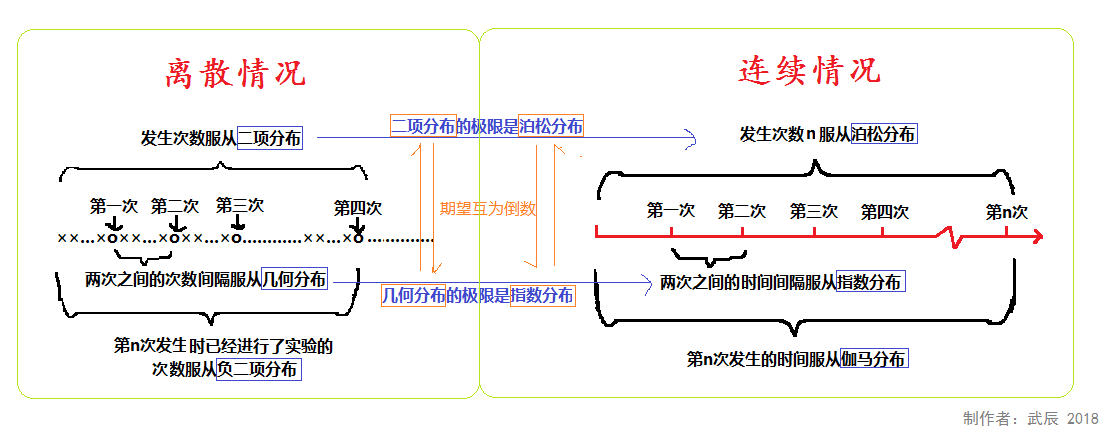https://www.jianshu.com/p/b570b1ba92bb
https://zhuanlan.zhihu.com/p/48140593
https://zhuanlan.zhihu.com/p/94181395
https://zhuanlan.zhihu.com/p/64859161
https://blog.csdn.net/touristman5/article/details/56281887
https://zhuanlan.zhihu.com/p/32932782


展开全文• 前言 ...本文主要整理概率密度函数（probability density function）和概率分布函数（probability distribution function）；主要针对连续型随机变量，也会稍微提及离散型随机变量。 概率密度函数 ...统计学
• ## 概率密度函数估计

万次阅读 2017-06-03 17:58:39
首先来看贝叶斯决策，贝叶斯分类器就是根据如下贝叶斯公式来设计的。最常用的就是比较后验概率的大小，进行类别决策。...这个呢就叫做类别的先验概率（类别就是男生、女生），对应公式上的p(w)。接着假设这个班参数估计 非参数估计 模式识别
• 最大熵方法与概率密度估计 ... 离散型随机变量的信息熵 考虑一个一维的离散的随机变量X（此处不考虑扩展到多维的情况），...考虑一个一维的连续型的随机变量X，若它的概率密度分布函数为f(x),那X在(a,b)之间的信息熵为：
• 学习笔记：数字图像处理——图像中噪声分布和概率密度函数的关系 学习的时候，看到各种噪声，高斯、锐利、伽马、均匀等。每个分布有概率密度函数，而这个和噪声有啥具体的连接的关系，我不是很懂，稍微研究了一下...噪声 噪声分布 图像处理 高斯噪声
• 1.ICA概念2.ICA不处理服从高斯分布的样本集3.概率密度函数4.复合函数的概率密度函数5.累积分布函数/分布函数6.联合分布7.行列式8.代数余子式(end)
• 使用Excel绘制F分布概率密度函数图表 利用Excel绘制t分布的概率密度函数的相同方式，可以绘制F分布的概率密度函数图表。 　F分布的概率密度函数如下图所示： 　其中：μ为分子自由度，ν为分母自由度 　Γ为...
• ## 高斯概率密度函数

千次阅读 2018-12-04 22:03:31
一维高斯PDF：   二维高斯PDF：   由此可以得到：。 如果用矩阵形式表示 对于联合高斯矢量 ，其PDF为：   其中均值...
• ## 二维概率密度求解边缘密度

万次阅读 多人点赞 2016-11-12 19:23:29
维概率密度求解边缘密度@(概率论)已知f(x,y)f(x,y)，求解fX(x),fY(y)f_X(x),f_Y(y)时，用的是下面的公式：fX(x)=∫+∞−∞f(x,y)dyfY(y)=∫+∞−∞f(x,y)dx f_X(x) = \int_{-\infty}^{+\infty}f(x,y)dy \\ f_Y(y) ...
•  在一般的高斯分布模型中，我们计算高斯分布概率密度函数p(x),回顾高斯分布的基本知识。通常如果我们认为变量 x 符合高斯分布 x~N(μ,σ2)则其概率密度函数为：，其中，μ,σ2分别表示如下：。 ...吴恩达
• 利用单位脉冲函数定义了离散型随机变量的概率密度,给出离散型随机变量与其独立的连续型随机变量和分布的计算公式,且证明其和分布不可能为正态分布。
• 在通信系统中，多径传播的包络一维分布为...典型案例是由同相分量和正交分量的联合概率密度函数一维包络和相位的联合概率密度函数，本文具体讲解雅各比行列式在概率密度函数坐标系转换中的应用，给出详细的证明过程。
• 比如，你想要从个服从正态分布的随机变量得到 100 个样本，那么肯定抽到接近其均值的样本的概率要大许多，从而导致抽到的样本很多是集中在那附近的。当然，要解决这个问题，我们通常都假设我们已经有了个 生成 0...随机数
• clear all; ...%%一维高斯函数 mu=0; sigma=1; x=-6:0.1:6; y=normpdf(x,mu,sigma); plot(x,y); figure; %%二维或多维高斯函数 mu=[0 0]; sigma=[0.3 0;0 0.35]; [x y]=meshgrid(linspace(-8,8,80
• 最大似然估计 有以下几个假设： 确定但未知 样本 独立同分布 类条件概率密度 有确定的函数形式 ...最大似然估计
• 维函数Z=g(X,Y)型，用卷积公式概率密度，积分区域如何确定（上） 因为关于二随机变量主题内容重要，难度大，例题多，最主要是积分区间的确定是难点，同时关联卷积概念，求二维函数Z=g(X,Y)型，用卷积公式求...
• 正态分布的概率密度和边缘分布(数1了解、数3掌握)三、第3章考研必做习题第3章习题：1、2、3、6、9、10、13、14、15、16、17、18、20第二节 边缘分布、边缘分布函数二、离散型随机变量的边缘分布律三、连...
• 维函数Z=g(X,Y)型，用卷积公式概率密度，积分区域如何确定（下） 因为关于二随机变量主题内容重要，难度大，例题多，最主要是积分区间的确定是难点，同时关联卷积概念，卷积公式容易，积分区间难以确定，因为...
• 它反映了观测样本xi对x处的概率密度估计的贡献，跟两个样本之间的距离有关，因此公式（2）的直观解释就是：对落入每个bin的所有观测样本的距离贡献值取平均，就会得到总体样本的概率密度函数估计。  对于核...非参数估计 直方图估计 kn近邻估计
• 因为关于二随机变量主题内容重要，难度大，例题多，最主要是积分区间...求二维函数Z=g(X,Y)型，用卷积公式概率密度，积分区域如何确定（中） #### ======= 【例二】 设二随机变量(X,Y)的概率密度为f(x...
• 联合概率密度 P(A^B) 条件概率 从面积比例看出，P(A|B)等于B中A的面积（P(A^B)）除以B的面积（P(B)）。 乘法公式（乘积法则） 假如事件A与B相互独立，那么： 相互独立：表示两个事件互不影响。 互斥：...
• 一元连续型随机变量及其概率密度 、联合概率密度函数 1.1、性质 1.2、例...